Technical Information Site of Power Supply Design

• TECH INFO
• Optimization of Frequency Characteristics of a DC/DC Converter in the Design Stage : Evaluating Frequency Characteristics to Check Output Stability and Response

Optimization of Frequency Characteristics of a DC/DC Converter in the Design Stage

# Evaluating Frequency Characteristics to Check Output Stability and Response

## -Part 1-

Keyword Output voltage stability and load transient response characteristicsDC/DC converter frequency characteristicsOutput responseLoad current transientOutput stabilityRingingOscillationFeedback circuitNegative feedbackNegative feedback becomes positive feedbackBode plotPhase delayPhase marginGain marginCrossover frequencyMoving toward greater stabilityResponsiveness is degradedTradeoff between stability and responseOptimization of stability and responseFrequency response analyzer (FRA)Modification of boards in actual equipment for the purpose of measurement is difficultSimulation

In DC/DC converter design, in order to finish with a power supply with satisfactory performance and specifications and with a circuit designed to run stably under actual conditions of use, in addition to static characteristics, dynamic characteristics must also be evaluated. Among these, output voltage stability and response are important points, and the frequency characteristics of the DC/DC converter must be understood in order to check and adjust them. In this article, Mr. Takayuki Atago was asked about the frequency characteristics of DC/DC converters and methods for their evaluation.

－To begin with, could you please explain the need for evaluating and understanding the frequency characteristics of a DC/DC converter.

This is likely the same for any design, but when desktop circuit design has ended and prototyping is performed, evaluations are conducted to determine whether design targets have been met. For DC/DC converters, in addition to confirming basic operation as a power supply such as the output voltage precision and the maximum output current, confirmation of the output voltage stability and load transient response characteristics is important. Stability and responsiveness are basically characteristics that are related to the designed DC/DC converter frequency characteristics, and therefore the frequency characteristics must be checked, and optimization must be performed based on the results.

－Could you explain output voltage stability and transient response in somewhat more detail?

Here is a diagram illustrating the different concepts, which I'll use in my explanation.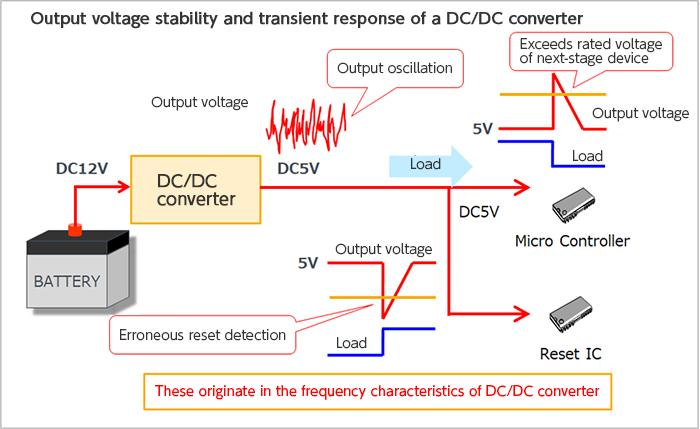I'll start with the responsiveness, which can be explained using this diagram alone. This schematic diagram is an example of a widely-used circuit in which the voltage from a 12-V battery is stepped down to 5 V by a switching type DC/DC converter, to be used as the power supply for a microcontroller. The 5 V voltage is monitored by a voltage monitoring IC, commonly called a reset IC, and if the voltage falls below a preset value, a reset signal is sent to the microcontroller.

Output voltage response means the action of returning to normal the output voltage when it has fluctuated momentarily due to a sudden change in the load current. Normally it is expected that a response will occur for as small a voltage fluctuation as possible, stabilizing the voltage at the preset voltage in as short a time as possible.

In the diagram, an example in which the load current increases suddenly and the 5 V output voltage momentarily falls, and an example in which, conversely, the load current decreases suddenly and the 5 V output voltage momentarily rises, are shown. When the drop in the output voltage caused by the sudden increase in the load current exceeds the reset IC threshold value, an unnecessary reset signal is sent, and the microcontroller is reset. And, when a sudden decrease in the load current causes the output voltage to rise sharply, exceeding the power supply voltage rating of the microcontroller, there are concerns that the microcontroller may malfunction or, in the worst case, undergo failure. This is something that needs to be checked without fail for the power supply of a device, such as a microcontroller or a CPU, which may be instantaneously woken from a sleep state and put into full operation, or may undergo the opposite transition.

By adjusting the transient response characteristics of a DC/DC converter, the device is optimized so that voltage fluctuations do not exceed the reset IC threshold value or the power supply rating of the next-stage device.

－I see. If the transient response characteristics are not optimized, critical problems could occur in some cases.

That's right. Now let's move to an explanation of output voltage stability. Here, output voltage stability means that excessive ringing and oscillation do not occur in the output. An example of the waveform of oscillation is shown. The output voltage from a DC/DC converter includes ripple voltages due to switching, and so is not completely a DC voltage; but oscillation is an anomaly different from ripple. This waveform diagram is an example of a DC/DC converter in a state in which oscillation occurs prominently when the load is removed.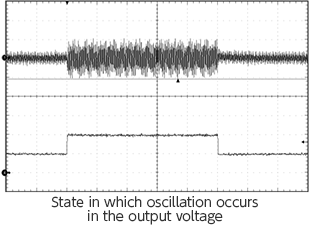－"Oscillation" brings to mind circuits that use op-amps. So oscillation occurs in DC/DC converters as well?

Oscillation in DC/DC converters is due to the fact that a feedback circuit is used to execute control in order to maintain a constant output voltage. Hence the principle of occurrence of oscillation and conditions are the same as for op-amps. It should also be noted that LDOs and other linear regulators also use feedback control, and so undergo oscillation under similar conditions. The principle of occurrence of oscillation is also connected to an understanding of frequency characteristics, so I will explain it in a bit more detail.

The internal circuit of a DC/DC converter IC is generally configured in this way. Points A and B, indicated by red arrows, are normally connected, but the signal B which is fed back for output control and the control signal A (output) based on the feedback signal, are intentionally separated.

The output voltage is fed back via voltage dividing resistors R1 and R2 to the error amplifier input within the IC. The error amplifier compares a reference voltage with the output voltage, and if the output voltage is higher than a preset voltage, executes control to lower the output voltage, but if lower, executes control to raise it, thereby maintaining a constant output voltage.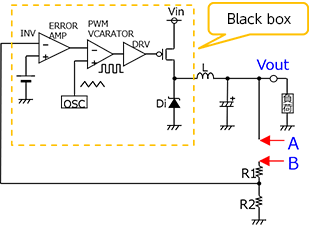Because negative feedback is used in feedback control, the phase of the control signal A corresponding to the signal B that is fed back is shifted by 180 degrees. The waveform diagram shows this phase relationship.

However, in actuality, a time delay always occurs since time is required for signal processing to be performed in the IC and a signal to be output. Hence as the frequency rises, the phase delay due to this delay time becomes greater. As the phase approaches zero degrees, an unstable state is entered, and if the phase delay reaches zero degrees, the negative feedback becomes positive feedback and anomalous oscillation occurs.－Is this the same as what is called an "insufficient phase margin" when oscillation occurs in an op-amp circuit?

It is essentially the same thing. I have been discussing only phase delay, but in actuality, stability should be examined using Bode plots, which are based on phase characteristics and gain characteristics.

－So a Bode plot, that is, the frequency characteristic, is connected to the stability and response of a DC/DC converter.

Yes. Now I'd like to explain the relationships between stability, response, and frequency characteristics in more detail. I'll proceed while summarizing what I've said so far.

Stability is "how much of a margin there is before oscillation conditions affect the output voltage," and response or responsiveness is "the response time from when the output voltage has fluctuated until it returns to the preset value." The phase margin, gain margin, and crossover frequency are parameters used to confirm these characteristics. These parameters can be read from a Bode plot generated for the DC/DC converter. The following diagram shows the relation of the Bode plot to each of these parameters and to stability and response.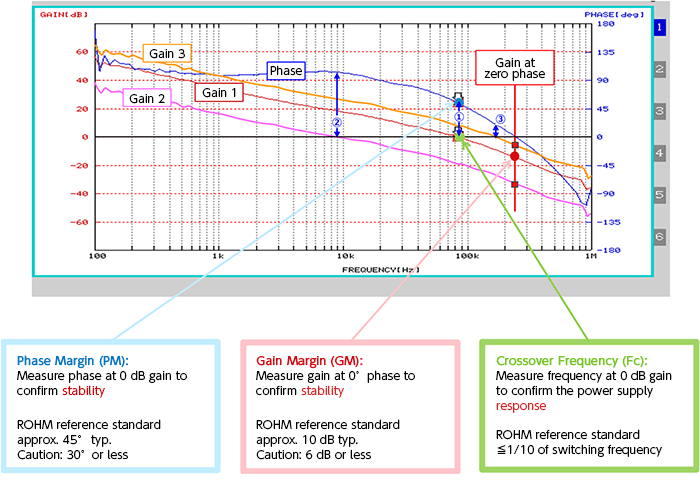I'd like to begin with this table, which summarizes measurements of these parameters, their relationships to stability and response, and corresponding tendencies for numerical values and characteristics. I'll then refer to this table to look at a Bode plot.

Phase margin Gain Margin Crossover frequency Phase at 0 dB gain Related to stability More stable when larger Gain at 0°(degree) phase Related to stability More stable when larger Frequency at 0 dB gain Related to response Response faster when higher

As indicated in the table and in the blue, red, and green boxes in the diagram, the phase margin is the phase at 0 dB gain, the gain margin is the gain at 0° (degrees) phase, and the crossover frequency is the frequency when the gain is 0 dB.

The phase margin and the gain margin are related to stability; the greater the value of either of these, the higher is the stability, and the less likely ringing or oscillation is to occur. The crossover frequency is related to response; the higher the crossover frequency, the better the responsiveness, and the more effectively output fluctuations due to load transients can be suppressed.

In the Bode plot shown, a curve (blue) representing the phase characteristic, and three types of gain characteristic curves (Gain 1: red, Gain 2: pink, Gain 3: orange), are plotted.

Gain 1 is a standard example. The frequency when gain 1 is 0 dB, that is, the crossover frequency, is about 85 kHz (the round green point), and the phase margin at that time is around 55 degrees (round light-blue point/blue double-arrow ①). The gain margin at phase 0 degrees is about 15 dB (round red point). In the explanations in the diagram, representative values for each of the parameters are indicated, and should be noted for reference.

Gain 2 is a hypothetical example for a case in which the gain is low. Because the gain is low compared with Gain 1, the crossover frequency has fallen to around 9 kHz, and the phase margin is above 100 degrees (blue double-arrow ②). The gain margin (the square red point on the Gain 2 curve) is 30 dB or greater, having increased together with the phase margin and moving toward greater stability. However, the crossover frequency has fallen, so that responsiveness is degraded.

Gain 3 is an example in which the gain is high. Because the gain is higher than in the case of Gain 1, the crossover frequency has risen to about 180 kHz, and the phase margin has dropped to around 20 degrees (blue double-arrow ③). The gain margin (square red point on the Gain 3 curve) has also fallen to 5 dB or so. In this example, because the crossover frequency is higher, responsiveness is improved, but because both the phase margin and the gain margin have fallen, stability is worsened.

As I think you've probably noticed, there is a tradeoff between stability and response; when one rises, the other falls. Hence in design, stability and response must be optimized in keeping with the circuit conditions.

－So I see that the frequency characteristic must be evaluated in order to check the stability and response of a DC/DC converter. How then does one acquire the frequency characteristic of a DC/DC converter?

To measure frequency characteristics, using a frequency response analyzer (FRA) is simple and reliable. An actual FRA and the measurement circuit are shown. The Bode plot we just saw was actually acquired using a FRA.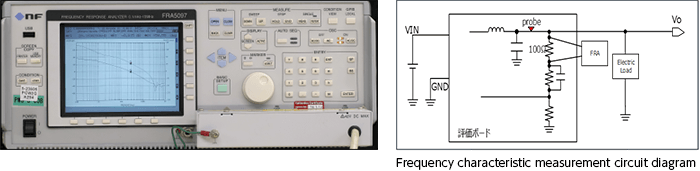To measure the frequency characteristic of a DC/DC converter, the feedback loop output and the voltage dividing resistors are separated, as in the measurement circuit diagram, a prescribed resistor (injection resistor) is inserted, and the FRA is connected to both ends of the resistor. Then, the phase margin and gain margin are measured more or less automatically, and the results are displayed on a graph.

－It seems fairly simple. When measuring actual equipment, is it necessary to modify the circuit board?

In fact, it is. It is true that an FRA can simplify measurement of the frequency characteristics, but that does not mean there are no issues to confront. The question you just asked is one such issue; and with the ongoing trends toward miniaturization and higher board densities, there will be not a few cases in which modification of boards in actual equipment for the purpose of measurement is difficult. Moreover, even if frequency characteristics are successfully measured, in order to make adjustments for optimization, the values of the relevant resistors and capacitors must be changed and the frequency characteristics measured again, and these tasks must be repeated. Similarly, small chip components on the boards of actual equipment must be replaced any number of times, and so a considerable amount of work is involved.

－In other words, in order to optimize frequency characteristics, trial and error involving repeated cycles of changing component values and performing measurements is necessary, and as components become smaller and board mounting densities rise, these are not simple tasks.

That's right. Moreover, there are many cases in which one won't have an expensive FRA available in the first place.

－Well, if one can't use a FRA, what other options are there?

Simulations are extremely useful, in the sense of supplementing actual measurements. Many recent simulators are capable of simulations of frequency characteristics and load response characteristics, and IC manufacturers provide device models and models including peripheral circuitry for use in simulations and the like.

－I certainly hear a lot about using simulations in design. Well then, I'd like to ask about simulations next.

＜to be continued＞